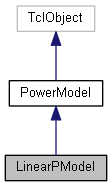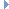GreenCloud Simulator
LinearPModel Class Reference

`#include <linearpmodel.h>`

Inheritance diagram for LinearPModel:[legend]
Collaboration diagram for LinearPModel:[legend]

## Public Member Functions

LinearPModel ()

virtual ~LinearPModel ()

virtual int command (int argc, const char *const *argv)

virtual double estimate (int size, double *predictors)

virtual double getMaxPower ()

virtual void print ()Public Member Functions inherited from PowerModel
PowerModel ()

virtual ~PowerModel ()

void setName (const char *name)

## Private Member Functions

void setCoefficient (const char *coef, double value)

void setCoefficientNumeric (const char *coef, double value)

void updateInit ()

void setCoefNumber (int number)

## Private Attributes

double * coefficients

bool * initialized

int coef_numberPublic Attributes inherited from PowerModel
std::string name_

## Detailed Description

Definition at line 13 of file linearpmodel.h.

## Constructor & Destructor Documentation

 LinearPModel::LinearPModel ( )

Definition at line 20 of file linearpmodel.cc.

20  {
21
22  /*LastResType+2 = +1 for elements number, +1 for the intercept:*/
23  coefficients = new double[LastResType+2];
24  initialized = new bool[LastResType+2];
26  for(int i = 0; i < LastResType+2; i++){
27  coefficients[i]= 0;
28  initialized[i]=false;
29  }
30
31 }
bool * initialized
Definition: linearpmodel.h:24
double * coefficients
Definition: linearpmodel.h:23
void setCoefNumber(int number)
Definition: linearpmodel.cc:40
 LinearPModel::~LinearPModel ( )
virtual

Definition at line 33 of file linearpmodel.cc.

33  {
34
35  delete[] coefficients;
36  delete[] initialized;
37  name_.clear();
38 }
bool * initialized
Definition: linearpmodel.h:24
double * coefficients
Definition: linearpmodel.h:23
std::string name_
Definition: powermodel.h:27

## Member Function Documentation

 void LinearPModel::addComponent ( DcResource * component )
virtual

Implements PowerModel.

Definition at line 52 of file linearpmodel.cc.

52  {
53  /* Linear power model does not accept components*/
54  return;
55 }
 int LinearPModel::command ( int argc, const char *const * argv )
virtual

Reimplemented from PowerModel.

Definition at line 128 of file linearpmodel.cc.

129 {
130
131  if (argc == 2) {
132  if (strcmp(argv, "print") == 0) {
133  /* print general info */
134  print();
135  return (TCL_OK);
136  }
137  } else if (argc==3){
138  if (strcmp(argv, "set-name") == 0) {
139  setName(argv);
140  return(TCL_OK);
141  } else if (strcmp(argv, "set-coef-number") == 0) {
142  setCoefNumber(atoi(argv));
143  return(TCL_OK);
144  } else {
145  return(TCL_ERROR);
146  }
147  }
148  else if (argc == 4) {
149  if (strcmp(argv, "set-coefficient") == 0) {
150  setCoefficient(argv,atof(argv));
151  return(TCL_OK);
152  } else if (strcmp(argv, "set-coefficient-numeric") == 0) {
153  setCoefficientNumeric(argv,atof(argv));
154  return(TCL_OK);
155  } else {
156  return(TCL_ERROR);
157  }
158  }
159  return (TCL_ERROR);
160 }
void setCoefficientNumeric(const char *coef, double value)
void setCoefficient(const char *coef, double value)
Definition: linearpmodel.cc:97
void setName(const char *name)
Definition: powermodel.cc:19
void setCoefNumber(int number)
Definition: linearpmodel.cc:40
virtual void print()
Definition: linearpmodel.cc:85
 double LinearPModel::estimate ( int size, double * predictors )
virtual

Implements PowerModel.

Definition at line 57 of file linearpmodel.cc.

57  {
59  if(size!= coef_number - 1){
60  std::cerr <<"Incorrect size of predictors array!\n";
61  }
62  double result = coefficients[size];
63  for(int i = 0; i < size; i++){
64  result += predictors[i] * coefficients[i];
65  }
66  return result;
67  } else {
68  std::cerr << "The model is not correctly initalized.\n" ;
69  print();
70  std::cerr << "Aborting simulation";
71  abort();
72  }
73 }
double * coefficients
Definition: linearpmodel.h:23
virtual void print()
Definition: linearpmodel.cc:85
 double LinearPModel::getMaxPower ( )
virtual

Implements PowerModel.

Definition at line 75 of file linearpmodel.cc.

75  {
76  double * load = new double[coef_number];
77  for(int i = 0; i < coef_number; i++){
79  }
81 }
virtual double estimate(int size, double *predictors)
Definition: linearpmodel.cc:57
 void LinearPModel::print ( )
virtual

Reimplemented from PowerModel.

Definition at line 85 of file linearpmodel.cc.

85  {
86  std::cout << "Linear model: "<< name_ << "\n";
88  std::cout << "Coefficients:\n";
89  for(int i = 0; i < coef_number ; i++){
90  std::cout << i << ": " << coefficients[i] << "\n";
91  }
92  } else {
93  std::cout << "Model not initalized properly\n";
94  }
95 }
double * coefficients
Definition: linearpmodel.h:23
std::string name_
Definition: powermodel.h:27
 void LinearPModel::setCoefficient ( const char * coef, double value )
private

Definition at line 97 of file linearpmodel.cc.

97  {
98  if(strcmp(coef, "Intercept") != 0){
99  res_type type = Resource::translateType(coef);
100  coefficients[type]=value;
101  initialized[type]=true;
102  } else {
103  coefficients[coef_number-1]= value;
104  initialized[coef_number-1]=true;
105  }
106  updateInit();
107 }
bool * initialized
Definition: linearpmodel.h:24
res_type
Definition: resource.h:19
static res_type translateType(const char *t)
Definition: resource.cc:85
double * coefficients
Definition: linearpmodel.h:23
void updateInit()
 void LinearPModel::setCoefficientNumeric ( const char * coef, double value )
private

Definition at line 109 of file linearpmodel.cc.

109  {
110  if(strcmp(coef, "Intercept") != 0){
111  int i = atoi(coef);
112  coefficients[i]=value;
113  initialized[i]=true;
114  } else {
115  coefficients[coef_number-1]= value;
116  initialized[coef_number-1]=true;
117  }
118  updateInit();
119 }
bool * initialized
Definition: linearpmodel.h:24
double * coefficients
Definition: linearpmodel.h:23
void updateInit()
 void LinearPModel::setCoefNumber ( int number )
private

Definition at line 40 of file linearpmodel.cc.

40  {
41
42  coef_number = number;
43  if(coefficients != NULL){
44  delete[] coefficients;
45  delete[] initialized;
46  }
47  coefficients = new double[coef_number];
48  initialized = new bool[coef_number];
49 }
bool * initialized
Definition: linearpmodel.h:24
double * coefficients
Definition: linearpmodel.h:23
 void LinearPModel::updateInit ( )
private

Definition at line 120 of file linearpmodel.cc.

120  {
121  bool result = true;
122  for(int i = 0; i < coef_number-1; i++){
123  result = result && initialized[i];
124  }
126 }
bool * initialized
Definition: linearpmodel.h:24

## Member Data Documentation

 int LinearPModel::coef_number
private

Definition at line 29 of file linearpmodel.h.

 double* LinearPModel::coefficients
private

Slopes as the resource types. The last element is the intercept

Definition at line 23 of file linearpmodel.h.

 bool* LinearPModel::initialized
private

Initialization flag

Definition at line 24 of file linearpmodel.h.